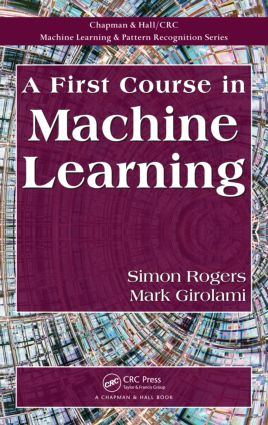# A First Course in Machine Learning

## 1st Edition

Chapman and Hall/CRC

305 pages | 124 B/W Illus.

##### Purchasing Options:\$ = USD
eBook (VitalSource) : 9781439892336
pub: 2011-10-28
from \$38.48

FREE Standard Shipping!

### Description

A First Course in Machine Learning covers the core mathematical and statistical techniques needed to understand some of the most popular machine learning algorithms. The algorithms presented span the main problem areas within machine learning: classification, clustering and projection. The text gives detailed descriptions and derivations for a small number of algorithms rather than cover many algorithms in less detail.

Referenced throughout the text and available on a supporting website (http://bit.ly/firstcourseml), an extensive collection of MATLAB®/Octave scripts enables students to recreate plots that appear in the book and investigate changing model specifications and parameter values. By experimenting with the various algorithms and concepts, students see how an abstract set of equations can be used to solve real problems.

Requiring minimal mathematical prerequisites, the classroom-tested material in this text offers a concise, accessible introduction to machine learning. It provides students with the knowledge and confidence to explore the machine learning literature and research specific methods in more detail.

### Reviews

"This book offers an introduction to machine learning for students with rather limited background in mathematics and statistics. … The book is well written and focusses on explaining themain concepts at a very basic level, keeping in mind the limited mathematical background of the intended audience. There are also useful references for further reading at the end of each chapter, and MATLAB code implementing the methods is available online along with the data sets. The code also seems to work well with free alternatives to MATLAB like Octave and FreeMat."

—Thoralf Mildenberger,IDP Institute of Data Analysis and Process Design, Zurich University of Applied Sciences, writing in Stat Papers (2015) 56:271

"… the authors do well to keep complicated mathematical notation of the kind sometimes found in statistical texts to a minimum. The concepts are introduced in quite a simple way so as to be intelligible to a reader with no statistical background. … this introductory text will be useful to computer scientists who need some basic introduction to statistical methods to apply in their respective problems …"

—Arindam Sengupta, International Statistical Review, 2014

### Table of Contents

Linear Modelling: A Least Squares Approach

Linear modelling

Making predictions

Vector/matrix notation

Nonlinear response from a linear model

Generalisation and over-fitting

Regularised least squares

Linear Modelling: A Maximum Likelihood Approach

Errors as noise

Random variables and probability

Popular discrete distributions

Continuous random variables — density functions

Popular continuous density functions

Thinking generatively

Likelihood

The bias-variance tradeoff

Effect of noise on parameter estimates

Variability in predictions

The Bayesian Approach to Machine Learning

A coin game

The exact posterior

The three scenarios

Marginal likelihoods

Hyper-parameters

Graphical models

A Bayesian treatment of the Olympics 100 m data

Marginal likelihood for polynomial model order selection

Summary

Bayesian Inference

Nonconjugate models

Binary responses

A point estimate — the MAP solution

The Laplace approximation

Sampling techniques

Summary

Classification

The general problem

Probabilistic classifiers

Nonprobabilistic classifiers

Assessing classification performance

Discriminative and generative classifiers

Summary

Clustering

The general problem

K-means clustering

Mixture models

Summary

Principal Components Analysis and Latent Variable Models

The general problem

Principal components analysis (PCA)

Latent variable models

Variational Bayes

A probabilistic model for PCA

Missing values

Non-real-valued data

Summary

Glossary

Index

Exercises and Further Reading appear at the end of each chapter.

### About the Author

Simon Rogers is a lecturer in the School of Computing Science at the University of Glasgow, where he teaches a masters-level machine learning course on which this book is based. Dr. Rogers is an active researcher in machine learning, particularly applied to problems in computational biology. His research interests include the analysis of metabolomic data and the application of probabilistic machine learning techniques in the field of human-computer interaction.

Mark Girolami is a chair of statistics and an honorary professor of computer science at University College London, where he is also the director of the Centre for Computational Statistics and Machine Learning. An EPSRC Advanced Research Fellow, an IET Fellow, and a Fellow of the Royal Society of Edinburgh, Dr. Girolami has made major contributions to the field, including his generalisation of independent component analysis, his work on inference in systems biology, and his innovations in statistical methodology.

### Subject Categories

##### BISAC Subject Codes/Headings:
BUS061000
BUSINESS & ECONOMICS / Statistics
COM000000
COMPUTERS / General
COM021030
COMPUTERS / Database Management / Data Mining